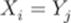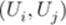﻿ ﻿Multiple DES - Data Encryption Algorithms - Introduction To Network Security: Theory And Practice (2015)

# Introduction To Network Security: Theory And Practice (2015)

### 2.3 Multiple DES

As discussed in Section 2.2.6, applying DES multiple times can effectively extend the length of encryption keys without modifying DES. Multiple DES can therefore be used to resist brute-force attacks. We useDES to denote a multiple DES scheme of applying DEStimes. By applying DES, it means applying either the encryption algorithmor the decryption algorithm.

2.3.1 Triple-DES with Two Keys

Triple-DES with two keys, denoted by 3DES/2, is the simplest and reasonably secure method against brute-force attacks. It extends the key length to 112 bits long. Letandbe two 56-bit encryption keys anda 64-bit plaintext block. The standard 3DES/2 encryption algorithm appliesonto obtain, then appliesonto obtain, and finally appliesonto obtain. That is,

2.7For convenience, we denote this scheme by.

The following is the 3DES/2 decryption algorithm:

2.8For convenience, we denote this scheme by.

We note that there are other combinations for 3DES with two keys, such asor. Any of these combinations would serve the purpose. However, only the combination ofallows us to use 3DES/2 to decrypt ciphertext string produced by applying single DES with key. This is done as follows: letand let. ThenA major drawback of 3DES is that its software executions are not as efficient as one would like them to be.

2.3.2 2DES and 3DES/3

In addition to 3DES/2, we may also apply DES twice with two keys, denoted by 2DES/2. For simplicity, we use 2DES to denote 2DES/2. Letandbe two DES encryption keys anda 64-bit plaintext block. The standard 2DES encryption algorithmand decryption algorithmare described as follows:However, 2DES is vulnerable to the meet-in-the-middle attack (see Section 2.3.3 for details). Thus, 2DES is considered nonsecure.

We may also apply DES thrice with three keys, denoted by 3DES/3. 3DES/3 has an effective key length of 168 bits. Let, andbe three encryption keys. The standard 3DES/3 encryption algorithmand decryption algorithmare described as follows:

2.92.102.3.3 Meet-in-the-Middle Attacks on 2DES

2DES is vulnerable to the meet-in-the-middle attacks. Suppose that the attacker has obtained two plaintext–ciphertext pairsand, whereThat is,The attacker may then be able to identify, with probability close to 1, the encryption keysandwith time complexity much smaller than. The attack can be carried out as follows:

List all 56-bit stringsand calculate, for each pair,Note that whenand, we have. Thus, for each pairwith, it is possible that. If there is only one such pair, then we have found the encryption keysand. Otherwise, apply each pairwithonto obtainAgain, we note that whenand, we have. Thus, if, thenis more likely to be the encryption key pair. Indeed, we can show that the possibility that there exist more than one such pair is very small.

Note that for any plaintext blockand any candidatefor the encryption key pair, the ciphertext blockis uniformly distributed (or close to being uniformly distributed). This is the property any good encryption algorithm should possess. Because, there arepairs. As, the expected number of pairsthat satisfyis or nearLikewise, the expected number of pairsfrom thesepairs that satisfyandis or nearThus, the possibility of findingis or near.

The time complexity of executing this attack is in the order ofThis is much smaller than.

﻿